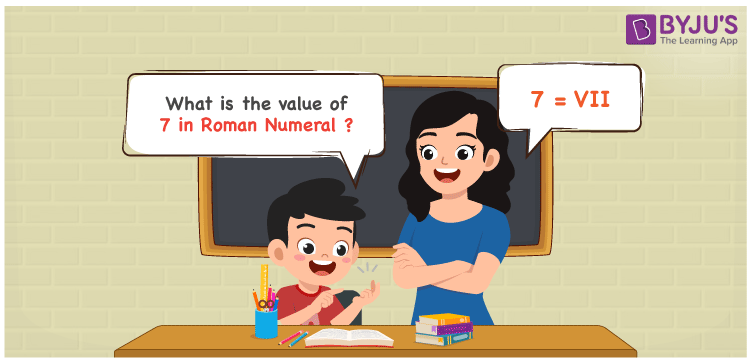# 7 in Roman Numerals

7 in Roman Numerals is VII. Roman numerals is an additive and subtractive system where letters are used to show certain numbers in the number system. A different combination of symbols are used to indicate the roman numerals that include the English alphabet. Therefore, the 7 can be written as VII in roman numerals.

 Number Roman Numeral 7 VII## How to Write 7 in Roman Numerals?

In order to write 7 in Roman Numerals, it has to be written in expanded form, i.e.

7 = 5 + 1 + 1

7 = V + I + I

7 = VII

## Video Lesson on Roman Numerals## Frequently Asked Questions on 7 in Roman Numerals

Q1

### Write 7 in Roman Numerals.

In Roman Numerals, 7 is written as VII.
Q2

### How to convert 7 into Roman Numerals?

The conversion of 7 into Roman Numerals includes breaking the number according to its place value.
Ones = 7 = VII
Number = VII
Q3

### What does 7 in Roman Numerals Mean?

To write 7 in roman numerals, we will first express 7 as the sum of numbers 5, 1, and 1, and further, write down their respective equivalent roman letters to apply the “additive notation”, i.e., 7 = 5 + 1 + 1 = V + I + I = VII. Hence, 7 in Roman numbers is expressed as VII.